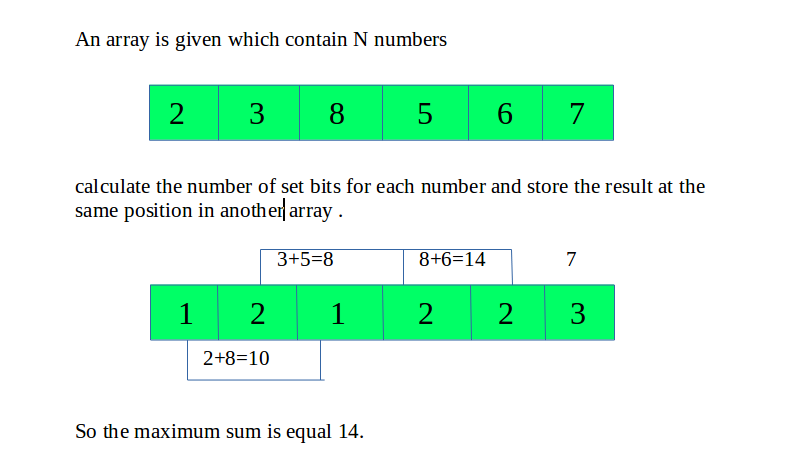Related Articles

# Maximum sum by adding numbers with same number of set bits

• Difficulty Level : Easy
• Last Updated : 21 May, 2021

Given an array of N numbers, the task is to find the maximum sum that can be obtained by adding numbers with the same number of set bits.
Examples:

Input: 32 3 7 5 27 28
Output: 34
Input: 2 3 8 5 6 7
Output: 14Approach

• Traverse in the array and count the number of set bits for every element.
• Initialize an array for 32 bits, assuming the number to have a maximum of 32 set bits.
• Iterate in the array and add the array element to the position which indicates the number of set bits.
• Traverse and find the maximum sum and return it.

Below is the implementation of the above approach:

## C++

 `// C++ program to find maximum sum``// by adding numbers with same number of set bits``#include ``using` `namespace` `std;` `// count the number of bits``// for each element of array``int` `bit_count(``int` `n)``{``    ``int` `count = 0;` `    ``// Count the number of set bits``    ``while` `(n) {``        ``count++;` `        ``n = n & (n - 1);``    ``}` `    ``return` `count;``}` `// Function to return the``// the maximum sum``int` `maxsum(``int` `arr[], ``int` `n)``{``    ``int` `bits[n];` `    ``// Calculate the``    ``for` `(``int` `i = 0; i < n; i++) {``        ``bits[i] = bit_count(arr[i]);``    ``}` `    ``// Assuming the number to be``    ``// a maximum of 32 bits``    ``int` `sum = { 0 };` `    ``// Add the number to the``    ``// number of set bits``    ``for` `(``int` `i = 0; i < n; i++) {``        ``sum[bits[i]] += arr[i];``    ``}` `    ``int` `maximum = 0;` `    ``// Find the maximum sum``    ``for` `(``int` `i = 0; i < 32; i++) {` `        ``maximum = max(sum[i], maximum);``    ``}` `    ``return` `maximum;``}` `// Driver code``int` `main()``{` `    ``int` `arr[] = { 2, 3, 8, 5, 6, 7 };``    ``int` `n = ``sizeof``(arr) / ``sizeof``(arr);``    ``cout << maxsum(arr, n);` `    ``return` `0;``}`

## Java

 `// Java program to find maximum sum``// by adding numbers with same number of set bits` `class` `GFG``{``// count the number of bits``// for each element of array``static` `int` `bit_count(``int` `n)``{``    ``int` `count = ``0``;` `    ``// Count the number of set bits``    ``while` `(n>``0``)``    ``{``        ``count++;` `        ``n = n & (n - ``1``);``    ``}` `    ``return` `count;``}` `// Function to return the``// the maximum sum``static` `int` `maxsum(``int``[] arr, ``int` `n)``{``    ``int``[] bits=``new` `int``[n];` `    ``// Calculate the``    ``for` `(``int` `i = ``0``; i < n; i++)``    ``{``        ``bits[i] = bit_count(arr[i]);``    ``}` `    ``// Assuming the number to be``    ``// a maximum of 32 bits``    ``int``[] sum=``new` `int``[``32``];` `    ``// Add the number to the``    ``// number of set bits``    ``for` `(``int` `i = ``0``; i < n; i++)``    ``{``        ``sum[bits[i]] += arr[i];``    ``}` `    ``int` `maximum = ``0``;` `    ``// Find the maximum sum``    ``for` `(``int` `i = ``0``; i < ``32``; i++)``    ``{` `        ``maximum = Math.max(sum[i], maximum);``    ``}` `    ``return` `maximum;``}` `// Driver code``public` `static` `void` `main (String[] args)``{``    ``int``[] arr = { ``2` `,``3` `, ``8``, ``5``, ``6``, ``7` `};``    ``int` `n = arr.length;``    ``System.out.println(maxsum(arr, n));` `}``}` `// This Code is contributed by mits`

## Python3

 `# Python3 program to find maximum``# sum by adding numbers with``# same number of set bits` `# count the number of bits``# for each element of array``def` `bit_count(n):``    ``count ``=` `0``;` `    ``# Count the number``    ``# of set bits``    ``while` `(n > ``0``):``        ``count ``+``=` `1``;` `        ``n ``=` `n & (n ``-` `1``);` `    ``return` `count;` `# Function to return the``# the maximum sum``def` `maxsum(arr, n):``    ``bits ``=` `[``0``] ``*` `n;` `    ``# Calculate the``    ``for` `i ``in` `range``(n):``        ``bits[i] ``=` `bit_count(arr[i]);` `    ``# Assuming the number to be``    ``# a maximum of 32 bits``    ``sum` `=` `[``0``] ``*` `32``;` `    ``# Add the number to the``    ``# number of set bits``    ``for` `i ``in` `range``(n):``        ``sum``[bits[i]] ``+``=` `arr[i];` `    ``maximum ``=` `0``;` `    ``# Find the maximum sum``    ``for` `i ``in` `range``(``32``):` `        ``maximum ``=` `max``(``sum``[i], maximum);` `    ``return` `maximum;` `# Driver code``arr ``=` `[ ``2``, ``3``, ``8``, ``5``, ``6``, ``7` `];``n ``=` `len``(arr);``print``(maxsum(arr, n));` `# This code is contributed by mits`

## C#

 `// C# program to find maximum``// sum by adding numbers with``// same number of set bits``using` `System;` `class` `GFG``{``// count the number of bits``// for each element of array``static` `int` `bit_count(``int` `n)``{``    ``int` `count = 0;` `    ``// Count the number``    ``// of set bits``    ``while` `(n > 0)``    ``{``        ``count++;` `        ``n = n & (n - 1);``    ``}` `    ``return` `count;``}` `// Function to return the``// the maximum sum``static` `int` `maxsum(``int``[] arr, ``int` `n)``{``    ``int``[] bits = ``new` `int``[n];` `    ``// Calculate the``    ``for` `(``int` `i = 0; i < n; i++)``    ``{``        ``bits[i] = bit_count(arr[i]);``    ``}` `    ``// Assuming the number to be``    ``// a maximum of 32 bits``    ``int``[] sum = ``new` `int``;` `    ``// Add the number to the``    ``// number of set bits``    ``for` `(``int` `i = 0; i < n; i++)``    ``{``        ``sum[bits[i]] += arr[i];``    ``}` `    ``int` `maximum = 0;` `    ``// Find the maximum sum``    ``for` `(``int` `i = 0; i < 32; i++)``    ``{``        ``maximum = Math.Max(sum[i], maximum);``    ``}` `    ``return` `maximum;``}` `// Driver code``static` `void` `Main()``{``    ``int``[] arr = { 2 ,3 , 8, 5, 6, 7 };``    ``int` `n = arr.Length;``    ``Console.WriteLine(maxsum(arr, n));``}``}` `// This Code is contributed by mits`

## PHP

 ``

## Javascript

 ``
Output:
`14`

Time Complexity: O(N * 32)
Auxiliary Space: O(N)

Attention reader! Don’t stop learning now. Get hold of all the important DSA concepts with the DSA Self Paced Course at a student-friendly price and become industry ready.  To complete your preparation from learning a language to DS Algo and many more,  please refer Complete Interview Preparation Course.

In case you wish to attend live classes with experts, please refer DSA Live Classes for Working Professionals and Competitive Programming Live for Students.

My Personal Notes arrow_drop_up